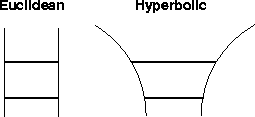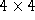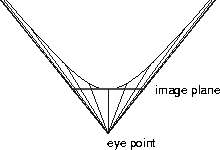Next: Implementation Up: H3: Laying Out Large Previous: Layout

# Hyperbolic Space

Our layout is computed using hyperbolic distances instead of the familiar euclidean distance measure. We use the hyperbolic metric in order to take advantage of the surprising property that hyperbolic space has more room than our familiar euclidean space. Two parallel lines are always the same distance apart in euclidean space. However, in hyperbolic space, parallel lines are not equidistant. We can construct two hyperbolic straight lines which do not intersect yet are separated by increasing distance as we move away from the origin. Figure 5 contains a sketch of both sets of lines. Further explanation of the ramifications of the hyperbolic metric can be found in one of the many mathematical textbooks which cover hyperbolic geometry [Mar75] [Wol45].Figure 5: Left: Parallel lines in euclidean space are always the same distance apart. Middle: In hyperbolic space the distance between two lines that never meet does indeed change. Here we show two geodesics which never meet but are not equidistant: the further they extend away from the origin, the more room there is between them.

When we deal with a single still image, a projection from hyperbolic space looks similar to a euclidean scene projected through a fisheye lens. However, motion of an object constructed with hyperbolic geometry is very different from the motion of a euclidean object. Although we could simply place euclidean objects into hyperbolic 3-space and move them around according to the rules of hyperbolic geometry, we would not be exploiting the exponential amount of room available in hyperbolic space.

## Projection

Although hyperbolic space is an infinite space more voluminous than euclidean space, we can project it into a finite volume of euclidean space. There are two standard projections which map all of hyperbolic space into a ball in euclidean space. The projective model preserves straight lines and distorts angles, while the conformal ball model preserves angles and warps lines. The 2D hyperbolic browser developed at Xerox PARC uses the conformal ball model [LRP95]. We use the projective model in our implementation and in the layout derivation in the Appendix. Transformations in the 3D projective model can be expressed asmatrices, so we use that model to gain maximum performance. The mapping from projective to conformal coordinates is straightforward, so our layout algorithm could be adapted for a conformal browser. A good discussion of hyperbolic transformations for use in computer graphics can be found in [PG92], so we do not discuss navigation details in this paper.

We show a diagram of a one-dimensional version of the projective model in Figure 5. We project from the hyperbola to a line segment tangent to the pole of the hyperbola that stretches between the asymptotes. Every ray projected from the hyperbola to an eye point at the crossing of the asymptotes will fall on this line segment, which is the image plane. In the one dimensional case, objects are line segments on the hyperbola. Objects near the pole of the hyperbola will be nearly undistorted. Projected rays for objects further away from the pole fall closer and closer together on the line segment. We thus see that rigid translations on the hyperbola result in shrinkage of the projected objects, which can never fall outside the line segment.Figure 6: An illustration of the projective model for one-dimensional hyperbolic space. The image plane is at the pole of one sheet of the hyperbola and the eye point is where the asymptotes meet. While the projection near the pole is almost undistorted, the apparent shrinkage increases as the rays reach further up the hyperbola.

In the three dimensional case we project from a volume to a volume. The analog of the line segment in 1D and the disc in 2D is the unit ball in three dimensional space. Our hyperbolic volume is a 3-hyperboloid, whose associated objects are the kind of three dimensional shapes that we usually see in 3D graphics. The same properties that we saw in the one dimensional case still hold. Objects at the center of the ball are undistorted. When we translate objects away from the pole of the 3-hyperboloid their projections grow smaller. These projections can approach, but never reach, the surface of the ball.

## Precision

Although the layout described in the Appendix uses hyperbolic distances, we must eventually project to from hyperbolic to euclidean coordinates in order to actually draw a picture with standard low-level graphics libraries. The time at which this conversion takes place has a major impact on the size of the static structure that can be displayed without encountering precision problems. Distinct hyperbolic coordinates which are too far from the origin will be projected so close to the surface of the unit ball that there are not enough bits to distinguish between their euclidean coordinates.

Objects that far from the origin do not need to be rendered, since they would project to an area much smaller than a pixel. The limit only comes into play if we store a static structure in euclidean coordinates that is much larger than the part which is actively being drawn.

In our implementation we do store a static structure: we project immediately after the layout phase, and then change the focus from one part of the structure to another by applying a transformation to the static structure. Nodes which are too far from the current root of the tree are marked as truncated, and can only be seen if the tree is laid out from a closer root. A more sophisticated implementation could defer the projection until render time and dynamically determine the appropriate euclidean coordinates for only objects in the neighborhood around the focus that are large enough to see.Next: Implementation Up: H3: Laying Out Large Previous: Layout

Tamara Munzner Tue Jul 22 08:42:41 PDT 1997